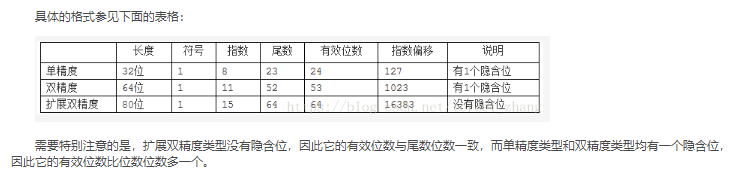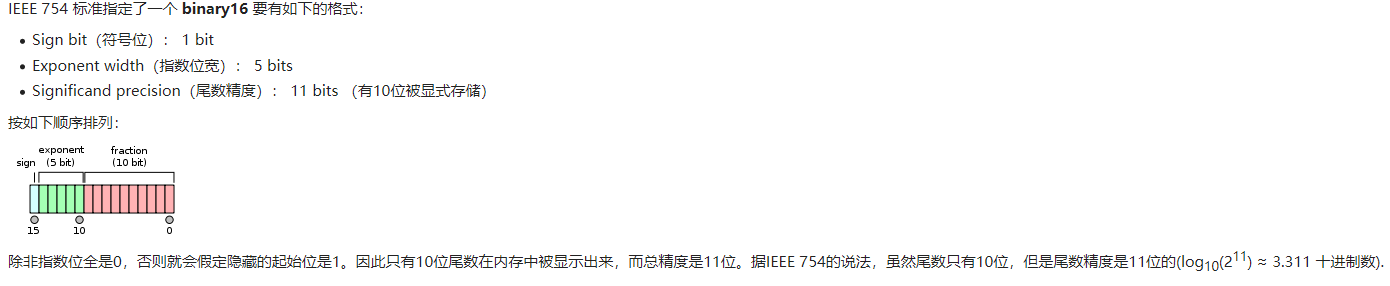• 126) * 0.1 = 2**(-127) 0 00000000 00000000000000000000001 = +1 * 2**(-126) * 0.00000000000000000000001 = 2**(-149) (Smallest positive value) 双精度 IEEE双精度浮点标准表示需要64位字，其可以从左到右表示...

注意：

Nintendo 64有一个64位处理器，但是：

Many games took advantage of the chip’s 32-bit processing mode as the greater data precision available with 64-bit data types is not typically required by 3D games, as well as the fact that processing 64-bit data uses twice as much RAM, cache, and bandwidth, thereby reducing the overall system performance.

The term double precision is something of a misnomer because the precision is not really double.

The word double derives from the fact that a double-precision number uses twice as many bits as a regular floating-point number.

For example, if a single-precision number requires 32 bits, its double-precision counterpart will be 64 bits long.

The extra bits increase not only the precision but also the range of magnitudes that can be represented.

The exact amount by which the precision and range of magnitudes are increased depends on what format the program is using to represent floating-point values.

Most computers use a standard format known as the IEEE floating-point format.

单精度

IEEE单精度浮点标准表示需要一个32位字，从左到右可以表示为从0到31编号。

>第一位是符号位，S，

>接下来的8位是指数位，’E’和

>最后23位是分数’F’：

S EEEEEEEE FFFFFFFFFFFFFFFFFFFFFFF

0 1 8 9 31

由字表示的值V可以如下确定：

>如果E = 255且F为非零，则V = NaN(“不是数字”)

>如果E = 255且F为零，S为1，则V = -Infinity

>如果E = 255且F为零，S为0，则V =无穷大

>如果0

意在表示通过用F加前缀而创建的二进制数

隐式前导1和二进制点。

>如果E = 0且F为非零，则V =( – 1)** S * 2 **(-126)*(0.F)。这些

是“非规范化”值。

>如果E = 0且F为零，S为1，则V = -0

>如果E = 0并且F为零并且S为0，则V = 0

尤其是，

0 00000000 00000000000000000000000 = 0

1 00000000 00000000000000000000000 = -0

0 11111111 00000000000000000000000 = Infinity

1 11111111 00000000000000000000000 = -Infinity

0 11111111 00000100000000000000000 = NaN

1 11111111 00100010001001010101010 = NaN

0 10000000 00000000000000000000000 = +1 * 2**(128-127) * 1.0 = 2

0 10000001 10100000000000000000000 = +1 * 2**(129-127) * 1.101 = 6.5

1 10000001 10100000000000000000000 = -1 * 2**(129-127) * 1.101 = -6.5

0 00000001 00000000000000000000000 = +1 * 2**(1-127) * 1.0 = 2**(-126)

0 00000000 10000000000000000000000 = +1 * 2**(-126) * 0.1 = 2**(-127)

0 00000000 00000000000000000000001 = +1 * 2**(-126) *

0.00000000000000000000001 =

2**(-149) (Smallest positive value)

双精度

IEEE双精度浮点标准表示需要64位字，其可以从左到右表示为从0到63编号。

>第一位是符号位，S，

>接下来的11位是指数位，’E’和

>最后52位是分数’F’：

S EEEEEEEEEEE FFFFFFFFFFFFFFFFFFFFFFFFFFFFFFFFFFFFFFFFFFFFFFFFFFFF

0 1 11 12 63

由字表示的值V可以如下确定：

>如果E = 2047且F为非零，则V = NaN(“不是数字”)

>如果E = 2047且F为零，S为1，则V = -Infinity

>如果E = 2047，F为零，S为0，则V =无穷大

>如果0

意在表示通过用F加前缀而创建的二进制数

隐式前导1和二进制点。

>如果E = 0且F为非零，则V =( – 1)** S * 2 **(-1022)*(0.F)

是“非规范化”值。

>如果E = 0且F为零，S为1，则V = -0

>如果E = 0并且F为零并且S为0，则V = 0

参考：ANSI / IEEE标准754-1985，二进制浮点算术标准。

展开全文• 基于FPGA双精度浮点运算器乘法模块的研究.pdf
• 当前NVIDA的GPU芯片仅支持单精度(float)浮点运算，对一些应用来说精度可能不够用，一些关键的步骤可能需要双精度运算，才能保证程序的正常执行。对此，本人尝试用两个单精度浮点数数来代表一个双精度浮点数：//类型...

当前NVIDA的GPU芯片仅支持单精度(float)浮点运算，对一些应用来说精度可能不够用，一些关键的步骤可能需要双精度运算，才能保证程序的正常执行。对此，本人尝试用两个单精度浮点数数来代表一个双精度浮点数：

//类型定义, 对于GPU编程，我们可以直接用内置类型float2, 这里的定义只是为了清晰起见。

typedef struct { float x, y;} float2

双精度和float2之间的转换

//双精度到float2的转换(有溢出的风险)

float2 double_to_float2(double val)

{

float2 ret;

ret.x = (float)val;

ret.y = (float)(val - (double)ret.x);

return ret;

}

//float2到双精度的转换

double float2_to_double(float2 val)

{

double ret;

ret = val.x;

ret += val.y;

return ret;

}

下一步就是关于 float2 类型的加减乘除的运算了(在GPU上实现)。说明，浮点运算中，括号是至关重要的；浮点数运算不满足结合律。

//加法： float2 + float2

static inline __device__ float2 add(float2 dsa, float 2 dsb)

{

float2 dsc;

float t1, t2, e;

t1 = dsa.x + dsb.x;

e = t1 - dsa.x;

t2 = ((dsb.x -e) + ( dsa.x - (t1 -e))) + dsa.y + dsb.y；

dsc.x = t1 + t2;

dsc.y = t2 -(dsc.x -t1);

return dsc;

}

//减法： float2 - float2

static inline __device__ float2 sub(float2 dsa, float2 dsb)

{

float2 dsc;

float t1, t2, e;

t1 = dsa.x - dsb.x;

e = t1 - dsa.x;

t2 = ((-dsb.x -e) + ( dsa.x - (t1 -e))) + dsa.y - dsb.y；

dsc.x = t1 + t2;

dsc.y = t2 -(dsc.x -t1);

return dsc;

}

#define split 8139.0

//乘法： float2 * float2

static inline __device__ float2 mul(float2 dsa, float2 dsb)

{

float2 dsc;

float a1, a2, b1, b2, cona, conb, c11, c21, c2, e, t1, t2;

cona = dsa.x * split;

conb = dsb.x * split;

a1 = cona - ( cona - dsa.x);

b1 = conb - ( conb - dsb.x);

a2 = dsa.x - a1;

b2 = dsb.x - b1;

c11 = dsa.x * dsb.x ;

c21 = (((a1*b1 - c11)+a1*b2)+a2*b1)+a2*b2;

c2 = dsa.x * dsb.y + dsa.y * dsb.x;

t1 = c11 + c2;

e = t1 - c11;

t2 = ((c2-e) + (c11 - (t1 -e))) + c21 + dsa.y * dsb.y;

dsc.x = t1 + t2;

dsc.y = t2 - ( dsc.x -t1);

return dsc;

}

//除法：  float2 / float2

static inline __device__ float2 div(float2 dsa, float2 dsb)

{

float2 dsc;

float a1, a2, b1, b2, cona, conb, c11, c2, c21, e, s1, s2;

float t1, t2, t11, t12, t21, t22;

s2 = 1.0f/dsb.x;

s1 = dsa.x * s2;

cona = s1 * split;

conb = dsb.x * split;

a1 = cona - (cona - s1);

b1 = conb - (conb - dsb.x);

a2 = s1 -a1;

b2 = dsb.x - b1;

c11 = s1 *dsb.x;

c21 = (((a1*b1 -c11)+a1*b2)+a2*b1)+a2*b2;

c2 = s1*dsb.y;

t1 = c11 + c2;

e = t1 - c11;

t2 = ((c2-e)+(c11-(t1-e))) + c21;

t12 = t1 + t2;

t22 = t2 - (t12 - t1);

t11 = dsa.x  - t12;

e = t11 -dsa.x;

t21 = ((-t12-e)+(dsa.x - (t11-e))) + dsa.y - t22;

s2 *= (t11+t21);

dsc.x = s1 + s2;

dsc.y = s2 - ( dsc.x - s1);

return dsc;

}

最后修改于 2008-10-28 10:35

阅读(?)评论(0)

展开全文• 3.浮点运算加法和乘法 3.1加法 3.2乘法 1.实数数的表示 参考深入理解C语言-03-有符号数，定点数，浮点数 1.1定点数 一般在没有FPU寄存器的嵌入式系统中使用比较多。比如常见的32位系统中，将高16位作为整数...

目录

1.实数数的表示

1.1定点数

1.2浮点数

2.精度说明

2.1半精度FP16

3.浮点运算加法和乘法

3.1加法

3.2乘法

1.实数数的表示

1.1定点数

一般在没有FPU寄存器的嵌入式系统中使用比较多。比如常见的32位系统中，将高16位作为整数部分，低16位作为小数部分。
这样就可以用整数来模拟定点数的 +  - * / 运算。
关于定点数的数学分析，请参考以下文档：
http://www.digitalsignallabs.com/fp.pdf

1.2浮点数

格式：

s符号位  exp指数   frac尾数   精度说明

16位       1            5             11            半精度 FP16
32位       1            8             23            单精度 FP32
64位       1           11            52            双精度 FP64
11位       1            4             6              没找到应用 11bit存储起来也奇怪

表示的数为： (-1)的s次方 *  2的(exp -base)次方 * (1 + frac)
base = 2的(exp位数 -1) -1 对于32位，为127 = 2的7次方 -1

比如0.325 =1.3 / 4  （规范化，这种方式在信息处理中很常见）
则s为0， exp为 127 + (-2) = 125, frac为0.3
近一步把0.3表示为  1/4 + 1/20 = (1/4) * ( 1 + 1/5)
注意到1/5可以表示为 (3/16) / (1 - 1/16) = (3/16) ( 1 + 1/16 + (1/16)*(1/16) + ... (无穷级数展开)
对应的二进制表示为
0.  01  (0011) (0011) ...
取前23位，则为：
0100110 01100110 01100110

这样，0.325在内存中的2进制表示为：
0  01111101  0100110 01100110 01100110

对应16进制为：3E A6 66 66

2.精度说明

半精度 16bit，单精度32bit，双精度64，上文已经提出，需要注意的是FP16，FP32，FP64都有隐藏的起始位。以半精度FP16为例说明

2.1半精度FP163.浮点运算加法和乘法

相比于整数加法和乘法多了比较，移位逻辑，比整数复杂很多

3.1加法

浮点加法器首先对浮点数拆分，得到符号、阶码、尾数。对拆分结果进行绝对值比较，得到大的阶码、阶差和比较结果输出。然后进行对阶，通过移位小的尾数，得到相同大阶。对尾数进行尾数加减运算，得到的结果进行规格化，最后结合规格化结果运算结果符号输出，得到结果输出。

一.需要注意一个是以绝对值大的对阶数(exponent), 二.对结果规格化规划到(1+Frac)的形式。

3.2乘法

符号位只是两者的异或，指数位基本上是两指数的相加，而尾数位就需要在正常的乘法之余考虑到移位（和随之而来的指数为的溢出）和进位的情况。

展开全文• MCU: STM32F767，启动硬件双精度浮点运算协处理器 IDE: Keil RVMDK V5.21.1.0 浮点协处理器：启用双精度浮点协处理器 主频：108MHz 测试方法 分别进行100万次双精度与单精度浮点数加运算，计算各消耗多少时间，打印...

测试平台

MCU: STM32F767，启动硬件双精度浮点运算协处理器
IDE: Keil RVMDK V5.21.1.0
浮点协处理器：启用双精度浮点协处理器
主频：108MHz

测试方法

分别进行100万次双精度与单精度浮点数加运算，计算各消耗多少时间，打印输出结果。

测试代码

void fpSpeedTest(void)
{
int begin_tick, end_tick;
double dp1 = 1.0;
double dp2 = 1.0;
double dp = 0.0;
begin_tick = HAL_GetTick();
for(int i = 0; i < 1000000; i++)
{
dp = dp1 + dp2;
}
end_tick = HAL_GetTick();
printf("double precision add 1000000 times used %d ms.\r\n", end_tick - begin_tick);
float fp1 = 1.0f;
float fp2 = 1.0f;
float fp = 0.0f;
begin_tick = HAL_GetTick();
for(int i = 0; i < 1000000; i++)
{
fp = fp1 + fp2;
}
end_tick = HAL_GetTick();
printf("single precision add 1000000 times used %d ms.\r\n", end_tick - begin_tick);
}

测试结果

double precision add 1000000 times used 112 ms.
single precision add 1000000 times used 197 ms.

结论

在开启 双精度浮点协处理器 的情况下，进行双精度浮点运算比单精度浮点运算消耗的时间更少，效率更高。不能仅凭直觉就认为单精度浮点运算精度低，运算速度当然更快。

展开全文• JS中使用IEEE-754规定的双精度浮点数，所有的算法也都符合IEEE-754规定的双精度浮点数运算双精度浮点数是一种64位(64 bit)的二进制数字格式。包括3个组成部分： 符号位 1位 阶码（指数部分） 11位 尾数（有效数...
• 浮点数(floating-point number)是属于有理数中某特定子集的数的数字表示，在计算机中用以近似表示任意某个实数。...位(bit)是衡量浮点数所需存储空间的单位，通常为32位或64位，分别被叫作单精度和双精度。...
• MCU: STM32F767，启动硬件双精度浮点运算协处理器 IDE: Keil RVMDK V5.21.1.0 测试方法 浮点数0.1分别以单精度与双精度累加1000000次，打印输出累加结果。 测试代码 double dVal = 0; float fVal = 0; for(int i ...stm32 c语言
• 实际上，任何精度浮点运算也可以像BigInteger那样计算，可以用多个浮点数据表示更高精度的浮点数据。而这就要求实现带余数的浮点四则运算。 带余数的浮点四则运算的定义： 加法和减法，余数和结果的和能精确表示...算法 c++
• 双精度浮点运算广泛应用于数值计算和信号处理中,在IEEE754标准中实现两个双精度浮点乘法需要一个53bit×53bit的尾数乘法器,这样的一个乘法器若采用FPGA实现需要大量的硬件资源。将Karatsuba算法应用于浮点运算器中,...
• 虽然说答案有点让人失望，但是在游戏显卡上FP64双精度浮点运算能力并没有什么用，实际上平常使用时很少用得上这东西，游戏是基本用不上的，日常的各种显卡加速功能也用不上，只有在模拟和财务分析时双精度浮点运算...
• 单精度浮点数与双精度浮点数区别 1、所占的内存不同 单精度浮点数占用4个字节（32位）存储空间来存储一个浮点数，包括符号位1位，阶码8位，尾数23位。 而双精度浮点数使用 8个字节（64位）存储空间来存储一个浮点数...编程语言 java
• 该楼层疑似违规已被系统折叠隐藏此楼查看此楼字换算计算机双浮点运算计算器初始数值：字数值：var button = window.document.getElementById("calculate");var result = window.document.getElementById("result...
• Python双精度浮点数运算并分行显示操作示例这篇文章主要介绍了Python双精度浮点数运算并分行显示操作,涉及Python数学运算及显示相关操作技巧,注释备有详尽的说明,需要的朋友可以参考下#coding=utf8def doubleType():...
• Cortex-M4PFU单元介绍近年，在Cortex-M3之后ARM公司又推出Cortex-M4内核，和之前的M3内核的区别之一就是M4带一个单精度浮点运算单元(PFU)。本文就FPU单元进行一个简单介绍，帮助工程师更快的理解FPU单元。1.Cortext-...
• 在C/C++中float是32位的，double是64位的，两者在内存中的存储方式和能够表示的精度均不同，目前C/C++编译器标准都遵照IEEE制定的浮点数表示法来进行float,double运算。 无论是float还是double，在内存中的存储...C 单精度 双精度
• 关于CPU的浮点运算能力计算

千次阅读 2020-12-22 11:27:46
只知道CPU主频不断的提升，核数也越来越多，但是如何理论上计算出不同代CPU性能的提升，还真没有认真思考过，我估计大多数人也不会关注，这次由于要进行高性能集群项目遇到，刚好认真的研究了一下CPU的浮点计算理论...
• 双精度浮点数double

千次阅读 2019-03-09 12:28:24
双精度浮点数（double）是计算机使用的一种数据类型。比起单精度浮点数，双精度浮点数(double)使用 64 位（8字节） 来存储一个浮点数。 它可以表示十进位制的15或16位有效数字。 sign bit(符号): 用来表示正负号 ...
• NVIDIA GPU的浮点计算能力

千次阅读 2020-01-13 14:15:52
​​其实说到浮点计算能力，首先得区分不同精度的浮点数，虽然Linpack测试里只关心双精度浮点计算能力，但在其他领域，有时候反而会更加关注单精度甚至是半精度的浮点计算能力。 半精度、单精度、双精度这些概念...
• 该工程代码实现了64位双精度浮点运算功能，所以的代码采用verilog 编写，附带测试脚本，以及进制转换工具。浮点运算器
• 单精度浮点数和双精度浮点数Here you will learn about difference between float and double i.e. float vs double. 在这里，您将了解float和double的区别，即float vs double。 These are two commonly used ...编程语言 java python jdk openssh
• Cortex-M4处理器的最大亮点之一，也是本文主要描述的一个功能就是增加了一个单精度浮点单元（FPU)，能够高效率处理较为复杂的浮点运算，如电机闭环控制、PID算法、快速傅里叶变换等。关于M4与M3的性能对比，官方提供...Cortex M4
• 双精度浮点型数据运算精度丢失以及数据的格式化问题
• STM32双精度运算

千次阅读 2013-11-28 22:03:48
调试程序的时候，用到两个双精度的运算，我写的公式比较长，这部分的计算竟然影响了中断部分的执行。具体点说，就是由于双精度运算，影响了进入和跳出中断...有些单片机有单独的浮点运算单元，估计那样会提高不少速度。
• 行业分类-物理装置-基于HXDSP芯片的双精度浮点复数矩阵运算优化方法.zip...